How to Union Pandas DataFrames using Concat?

• Difficulty Level : Medium
• Last Updated : 10 Jul, 2020

concat() function does all of the heavy liftings of performing concatenation operations along an axis while performing optional set logic (union or intersection) of the indexes (if any) on the other axes.

The concat() function combines data frames in one of two ways:

• Stacked: Axis = 0 (This is the default option).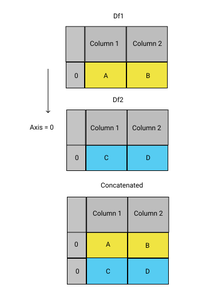Axis=0

• Side by Side: Axis = 1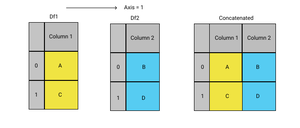Axis=1

Steps to Union Pandas DataFrames using Concat:

• Create the first DataFrame

Python3

 import pandas as pd  students1 = {'Class': ['10','10','10'],            'Name': ['Hari','Ravi','Aditi'],            'Marks': [80,85,93]           }  df1 = pd.DataFrame(students1, columns= ['Class','Name','Marks'])  df1

Output: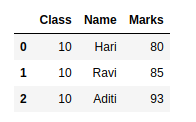• Create the second DataFrame

Python3

 import pandas as pd  students2 = {'Class': ['10','10','10'],            'Name': ['Tanmay','Akshita','Rashi'],            'Marks': [89,91,87]           }  df2 = pd.DataFrame(students2,                    columns= ['Class','Name','Marks'])  df2

Output: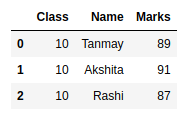• Union Pandas DataFrames using Concat

Python3

 pd.concat([df1,df2])

Output: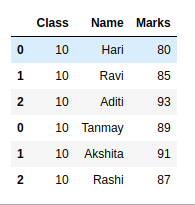Note: You’ll need to keep the same column names across all the DataFrames to avoid any ‘NaN’ values.

My Personal Notes arrow_drop_up